Data Science Interview Questions for IT Industry Part-5: Reinforcement ML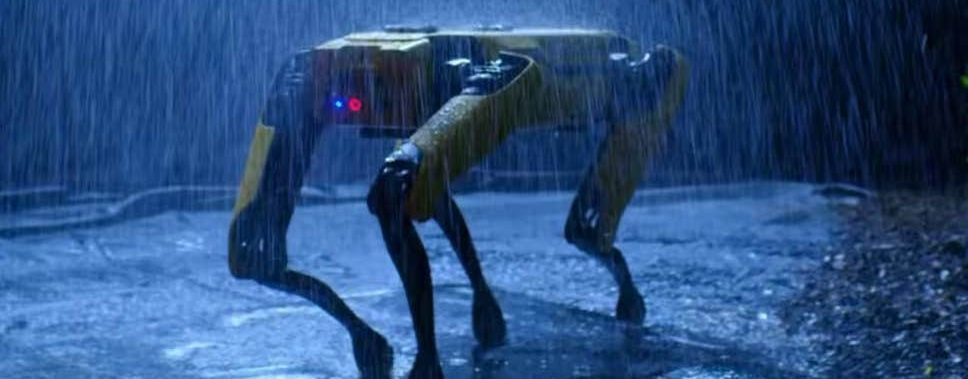In the previous post, I talked about the data science interview questions related to various algorithms under unsupervised machine learning. In this post, I will discuss the questions and algorithms related to Reinforcement machine learning, which currently holds the key to the future of AI.

Q. What is Reinforcement Learning in simple words?

Reinforcement Machine Learning is a branch of Machine Learning where the algorithm performs a certain action on its own, evaluates the result of the action, and then decides what to do next, keeping the end goal in mind.

It can be said that reinforcement learning is true Artificial Intelligence! Here, we just define a set of rules and leave the decision making to the algorithms, hence, making them “autonomous”.

To understand this better, let’s take a real-life scenario.

When you try to warn a kid to not touch fire because it will hurt, you are “supervising” the learning of the kid. This is supervised machine learning.

When the kid tries to touch the fire anyway and jumps back due to the burning sensation. The kid(agent) is interacting with the fire(environment) and learns that it is not good to touch fire.

Hence, the kid is not going to touch the fire again due to the pain(penalty) experienced and decides the next action “stay away from fire” in order to be safe and happy(reward). This is Reinforcement Machine Learning.

In fact, if you look back at your lives, you can see in hindsight that you have been doing this all the way along! Interacting with the environment(Life), making mistakes(bad choices), taking feedback(consequences/rewards), and deciding the next course of action based on the experiences.

We are all agents interacting with the environment continuously, trying to maximize the rewards without the knowledge of the future!

The above can be summarized in this diagram The agent interacts with the environment, chooses the best available next action based on the feedback from the environment.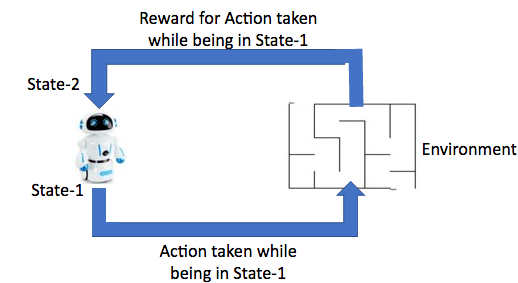Reinforcement Learning: The agent takes some action while being in a state say “State-1”. Hence, the environment gives back the associated reward and takes the agent to the next “State-2”

Q. What is an agent?

An agent is an entity(Human/robot/algorithm) that interacts with its environment(Life/maze/problem) and gets feedback(reward/penalty) from the environment for every action. Based on the feedback, the agent decides which action to take next while keeping the end goal in mind.

For example, if a robot needs to deliver food to table-10, then then it has to find the safe path(policy) from the kitchen to the table-10 on its own.

The restaurant space is the environment, the robot is the agent, the best path is known as policy, spilling the food is a penalty, keeping food safe is the reward and delivering the food to table-10 is the end goal.

Q. What is the environment?

The environment is the place where the agent operates. It could be physical(Restaurant/roads/rooms) or non-physical (virtual maze/tree traversal) as well. It contains multiple states (positions).

The environment provides feedback to the agent for its actions. If the action in any state is in-line with the end goal, then the agent is rewarded otherwise penalized. When the agent reaches the final state (end goal) then the environment sends a termination signal which stops the agent from performing further actions.

Q. What is the State?

A State is a location in the environment. These are of three types

• Initial State
• Intermediate States
• Final State

The objective of any agent is to start at the Initial State, choose the best combinations of Intermediate States(Policy) in order to reach the Final State.

Every state has a limited set of states reachable from it. If the agent chooses a reachable state then reward is given. However, if the agent chooses an unreachable state then it is penalized.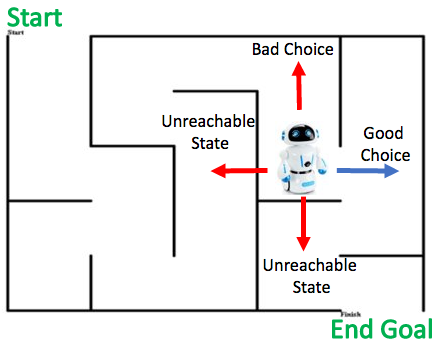The agent chooses to move to the next state from the current state based on the reward and penalties

For example, in the above diagram, the agent(Robot) can choose to move in any of the four directions in the current state.

However, there is only one move which is good i.e. to move the right side. The reverse movement is a bad choice since it takes the agent away from the end goal.

The other two moves are impossible due to the wall, hence, these states are not accessible to the agent. if the agent chooses to move to these sides then the environment will penalize the agent.

Once the agent reaches the final state, a stop signal is sent from the environment to the agent, which ends the current episode.

Q. What is the policy?

A policy is the “mindmap” of an agent which helps it to decide what action to take in which state.

Basically, a guide for the agent which tells what action to take in a given state so that it leads to the end goal while earning the highest possible rewards on the way. A policy is denoted by π

The whole idea of reinforcement learning is that the agent finds an optimal policy that leads it to the end goal(final state) with maximum rewards.

In other words, a policy is the best possible path from the initial state to the final state. Mathematically, the best policy has the highest amount of rewards associated with it.

The whole idea of reinforcement learning is that the agent finds an optimal policy that leads it to the end goal(final state) with maximum rewards.

In the above diagram, the objective for the agent is to reach the end goal of the maze via the shortest path.

The agent has to figure out this shortest path(Best Policy) based on its interaction with the environment(Maze) while keeping the target in mind. There is a chance of taking a wrong path and then returning back from the dead end. This happened in Policy-1, which resulted in a penalty by the environment to the agent, hence the reward got reduced.

Policy-2 is the best possible path, with no mistakes, hence it maximizes the reward.

Q. What is a reward?

The reward is a way the environment talks with the agent.

If the agent performs a good action then the environment sends a high reward signal otherwise a low reward signal(penalty) is sent.

The amount of reward depends on the goodness of the action. If the agent performs a good action, then the reward is more. A good action is the one that leads the agent towards the end goal in the best possible way.

Q. What is an episode?

One full cycle of an agent beginning at the initial state and reaching the final state. Once the agent reaches the final state, the environment sends a terminal signal which asks the agent to stop.

Q. What is a Markov Decision Process?

A Markov Decision Process(MDP) is a mathematical framework to code sequential decision making by algorithms on its own, by means of reward signals. The actions of the agent are influenced by below two factors

• How good the current action is based on the current reward?
• How this action will affect all future actions and cumulative rewards?

MDP is the ideal theoretical form of Reinforcement Learning. There are five main components of an MDP listed below. These put together, represent a way to describe a sequential decision-making process, where the control is partially stochastic(random) and partially under the decision-maker(agent).

• State
• Action
• Transition Function (Old State to New State, Action)
• Reward Function (State, Action)
• Policy

The goal of an agent is to find the best policy(strategy of taking action) based on the learnings from the environment while interacting with it.

The interaction of an agent with the environment happens in the below steps…

1. The agent is present in a starting state(s0)
2. It chooses to take one of the available actions(a1 or a2 or a3) in the current state(s0)
3. Based on the action chosen in the above step, the environment gives back a reward(R1 or R2 or R3) using the Reward Function. Some rewards will be high and some will be low based on the environment.
4. The Agent will move to the next state(s1 or s2 or s3) based on the action taken using the state Transition Function
5. Repeat step1-step4 until the environment sends a termination signal.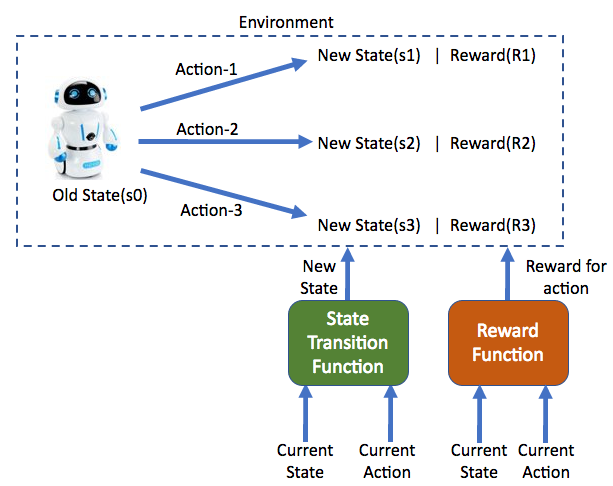In a Markov Decision Process, the agent takes an action(a1/a2/a3) while being in a state(s0) and receives the corresponding reward for it while moving to the new state(s1/s2/s3).The values of reward and the new state are governed by a Reward Function and a State Transition Function respectively

Q. What are the types of Reinforcement Learning?

There are two major types of Reinforcement Learning algorithms.

• Model-Based
• Model-Free

Here, the term model can be very confusing! Because we are coming from a supervised and unsupervised machine learning background. In the context of Reinforcement Learning, a model is a combination of below two functions which dictates the behavior of the environment. Hence we can say that it is a model of the environment.

1. State Transition Function: If an agent is present in a state(s1) and takes an action(a1), then what is the probability that the agent will move to the next state(s2).
2. Reward Function: If an agent is present in a state(s1) and takes an action(a1), then what will be the reward returned by the environment.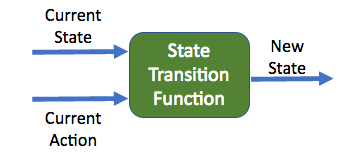A State Transition Function takes an input of the current state and the action performed. Based on this it gives the output of the next state to go.

Model-Based Reinforcement Learning algorithms use the above two functions to find the best policy. e.g. an Markov Decision Process and Dynamic Programming.

Model-Free Reinforcement Learning algorithms DO NOT use these functions. Instead, they interact(trial and error) with the environment to find the best policy. e.g. Monte Carlo methods and Temporal Difference methods.

Think of these functions as a navigation device of the environment! If these are present, then the agent knows what action will take it to which state even before interacting with the environment!

Like we get to know which roads are currently having heavy traffic using google maps even before hitting the road! Hence, we decide the best path(Policy) to reach our destination.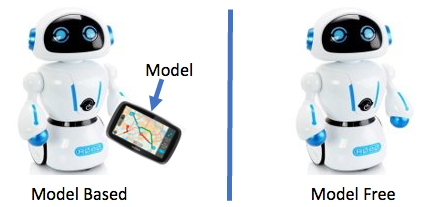Model-Based algorithms know how to navigate an environment even before interacting with it. Whereas Model-Free algorithms learn to navigate the environment by interacting with it!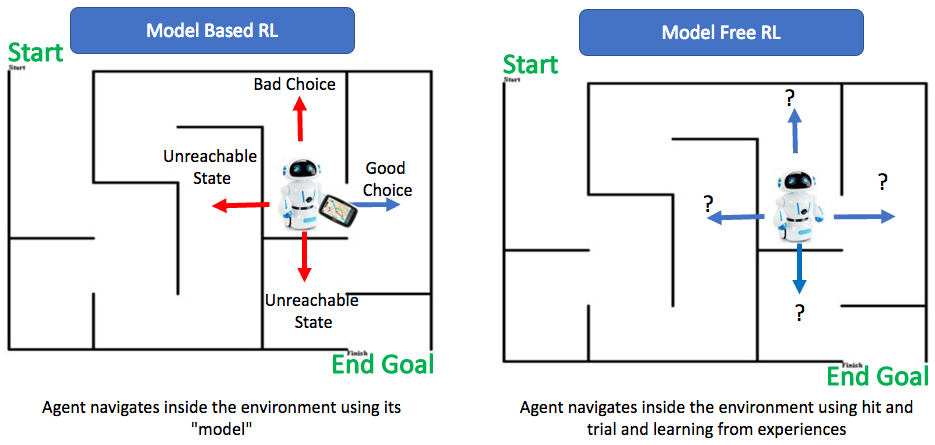In the left figure, the agent is holding a “model” in its hand which is helping it to choose the best action in every state.

In the context of Reinforcement Learning, a model is a combination of Reward and State Transition functions which dictates the behavior of the environment.

Why should we do trial and error at all? Why can’t we just use these functions?

In most of the Reinforcement Learning problems, the Reward and State Transition functions are NOT available, i.e. the agent does NOT know how the environment will react if it takes an action(a1) in a state(s1). Hence, we have to use the model-free approach.

Q What is Return?

The sum of rewards which is expected in the future if the current policy is followed by the agent after timestamp “t”. It is denoted by “Gt“.

In the most simple case, it can be computed with the below equation. If the agent is at a time step “t” then the expected future return is the sum of all the rewards after time step “t”.
“T” represents the final time step.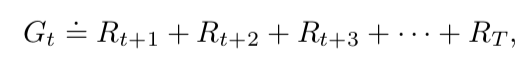At timestamp “t” the expected return in the future is the sum of all the rewards which the agent will get in its lifetime

There is a problem with this equation if T is not limited. The agent can keep taking some actions and keep receiving some rewards forever, which will make “Gt” infinite!

Hence, to keep the time in mind, let us reduce the value of reward if it is received after a very long time so that the agent will try to complete its task as early as possible.

This can be done by multiplying all future rewards after time step t+1 by a shrinking factor called “discount rate“.

The discounting is denoted by gamma(γ) and it can take values between 0 and 1. Now, the agent is present at time step “t” and is trying to maximize the sum discounted rewards “Gt” from all the future time steps. Allowed values of gamma are between 0 ≤ γ ≤ 1.

Q. What is Value?

Rewards give a short term view of good actions, Value gives the long term view of good actions to the agent.

The Value of a state is the sum of all the rewards the agent can expect in the long run.

It helps the agent to evaluate its next action. The agent can perform the analysis like “If I take this action from the current state and reach the next state, how good this will be in the long run?”

Sometimes an action can give high reward immediately but reduce overall rewards afterward due to states which will come up next. The Value of a state helps the agent keep an eye towards the end goal.

If an agent is following a particular policy in a state which will lead it to the end goal with maximum rewards, the value of the current state will be high since it leads to the end goad via a path(policy) of high rewards.

While being in a given state, the agent can iterate through different policies(strategy/path) to “see” which one is offering the highest rewards from the current state and start using that policy

Almost all the reinforcement learning algorithms use the concept of Value to find out the best policy. Value is calculated in two ways

Value of a state at a given time step

It is defined as the expected return while starting in a state s and following the policy π after that. It is denoted as Vπ(s) and called state-value function for policy π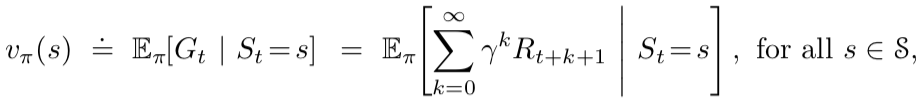State value function evaluates the expected return of a state “s” if a policy “π” is followed after it

Value of a state at a given time step if an action “a” is performed

It is defined as the expected return of taking an action “a” in a state “s” and following the policy “π” after that.  It is denoted as qπ(s, a)  and called action-value function for policy π.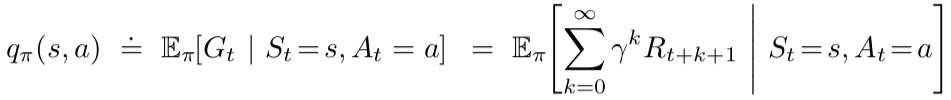The action-value function evaluates the expected return of a state “s” if an action “a” is taken and policy “π” is followed after it

The agent tries to create an approximation of these functions (s) and qπ(s, a) by tuning the parameters while matching the actual reward values from the environment at each time step.

Q. What is Dynamic Programming?

When the model of the environment is known … as it is known for the Markov Decision Process(MDP), then we can find the optimal policy using the model(state transition and reward functions). This is known as dynamic programming.

Dynamic programming is practically not scalable due to the high amount of processing power needed for simple environments with few states also, this is because we have the perfect model of the environment hence, every possible action and the result of that action can be evaluated to find the best policy. This can lead to a very high number of scenarios that need to be evaluated.

This is the reason model-free techniques like Temporal Difference and Monte Carlo are preferred over Dynamic Programming for all practical Reinforcement Learning problems.

There are four major algorithms under Dynamic Programming listed below.

• Policy Evaluation
• Policy Improvement
• Policy Iteration
• Value Iteration

Q. What is Policy Evaluation?

Policy Evaluation is a Dynamic Programming technique. It finds the optimal value function “Vπ *” for a given policy “π” by iterating over different value functions possible and finding out the returns given by each of them. In the end choosing that value function which gives maximum returns.

Q. What is Policy Improvement?

Policy Improvement is a Dynamic Programming technique. It finds the optimal policy “π *” for a given value function “Vπ” by iterating over all possible policies and selecting the one which generates maximum rewards. Hence, Improving the policy outcome for the given value function.

Q. What is Policy Iteration?

Policy Iteration is a Dynamic Programming technique that combines the power of policy evaluation and policy improvement together.

The algorithm can be summarised by the below steps.

1. Given a Policy “π“, use Policy Evaluation to find out the optimal value function”Vπ *” for it.
2. Once the optimal value function is found, use Policy Improvement to find the optimal policy “π *” for it.
3. Repeat step-1 and step-2 till no further improvement is seen in the expected returns.

Q. What is Value Iteration?

Value Iteration is a Dynamic Programming technique that improvises policy iteration algorithm by cutting short every evaluation step to just one sweep. By restricting policy evaluation to stop after one round, the Value Iteration algorithm saves a lot of time and helps in faster convergence(Finding optimal policy).

This is feasible because, after the first round of policy evaluation, there is no significant change in the value function, hence we can stop searching for the optimal value function and proceed with the next step of policy improvement.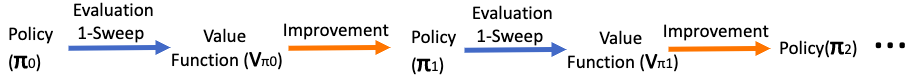Value Iteration: Using Policy Evaluation and Policy Improvement recursively till optimality is reached but restricting Policy Evaluation to single sweep each

Q. How do Monte Carlo algorithms works?

Monte Carlo techniques are one of the ways to solve a reinforcement learning problem when there is no model available for the environment.

The core idea behind Monte Carlo algorithms is to interact with the environment multiple times and take the average of returns observed at each state during each interaction(episodes).

Monte Carlo algorithms are applicable only to episodic tasks, which means the interaction with the environment will come to an end for sure after some time, also known as the end of an episode.

After many episodes(interactions with the environment) are finished, the algorithm takes the average of the return values of each state to create an action-value function qπ(s, a), which helps the agent to navigate through the environment and find an optimal policy(π*)

The below diagram represents a sample environment and three Monte Carlo episodes. Here S1/S2 is the starting state and S7 is the final state. The agent has to find the best policy(path) from S1 to S7.

As I stated, Monte Carlo learns by experiences from multiple episodes and takes the average of these episodes. If the value of any state is to be calculated, then all we have to do is to take the average of the rewards received after it from all the episodes.

Let us calculate the Value of state “S4”

Value(S4)=AVG ( Value(S4) in Episode1, Value(S4) in Episode2, Value(S4) in Episode3 )

Value(S4)=AVG ((10+5) + (10+5) + (15-10+10+5+5))

Value(S4) = 18.33

Similarly, the value of any state can be computed using the data from multiple episodes.

Now, you might have observed that in Episode-3, the state S4 was visited twice. But, the returns were calculated based on the first occurrence only. This is known as First visit Monte Carlo.

If we calculate the returns of a state by taking the average of the returns received after each visit of that state from all the episodes, then it is known as the Every visit Monte Carlo.

Q. What is Monte Carlo Prediction?

The estimation of state-value function(V) or action-value function(q) is known a prediction problem in reinforcement learning.
As discussed in the previous section, Monte Carlo finds out value for a given state by averaging the returns of that state in multiple episodes.

Since a state can be visited more than twice in a single episode.
The value for a state can be computed in two ways:
1. First Visit: Average the returns after the first visit to the given state. known as First Visit Monte Carlo.
2. Every Visit: Average the returns after every visit to the given state. known as Every Visit Monte Carlo

Q. What is the problem of maintaining exploration?

While estimating action-value function, various state-action pairs are visited and the returns are averaged for multiple episodes.
What if… certain state-action pairs are never visited even if we conduct infinite episodes? Then the returns for those states cannot be computed since we don’t have any interaction data. This is known as the problem of “maintaining exploration“.

This is solved by assigning a non-zero probability to each state-action pair to get selected. Hence, making sure that each state-action pair will get selected definitely… if an infinite number of episodes are run.
This assumption is also known as the assumption of “exploring starts”. It means that the algorithm will definitely visit all possible state-action pairs in the course of infinite episodes.

Q. What is Monte Carlo control?

The estimation of the optimal policy is known as the control problem. Basically finding the rules which “controls” the movement of the agent inside the environment. These rules are known as a policy.

Monte Carlo control uses the generalized policy iteration(GPI) to find out the best policy.
1. Select a random policy P1
2. Perform policy Evaluation of policy P1 to find its best action-value function q1
3. Use the optimal action-value function q1 and find do poliy iteration to find the best policy P2 for the q1
4. Repeat steps 2 and 3 till optimal policy p* and optimal action-value function q* is found.

Q. What is On-policy vs Off policy methods?

On-Policy methods:
Improve the same policy which is being used currently.

Off-Policy methods:
One problem with the On-Policy method is that it never really finds the optimal policy since it is always exploring. This is solved using Off-Policy method.
A simple solution is to use one policy to explore the behavior of the environment and generate the data known as behavior policy. Use this data to improve another policy which is called the target policy.

The trick here is to keep behavior policy “soft” and target policy “greedy”.
This ensures that the behavior policy explores the environment(soft policy) and tries new actions while the target policy uses the learnings from behavior policy and finds optimal policy by being greedy and choosing only those actions which give back maximum value.

Q. What is importance sampling?

The estimation of expected values in one distribution based on the samples from another distribution is known as Importance Sampling. It is used in Off-Policy Methods.
There are two types of importance sampling.
1. Ordinary importance sampling: Use the simple average of samples
2. Weighted importance sampling: Use the weighted average of samples

Q. What is Temporal Difference(TD) Learning?

Temporal Difference Learning is the most extensively used method for implementing reinforcement learning. If there is one idea which can be linked behind true Artificial Intelligence, then it will be the Temporal Difference.

Popular Temporal Difference algorithms are SARSA and Q-Learning.

The naming convention is based on the way this method works. By updating the action-value/state functions based on the value/reward differences of current timestamp “t” and the next timestamp “t+1“.

Temporal Difference is the next level of reinforcement learning which combines and improves the ideas of Monte Carlo and Dynamic Programming

Temporal Difference is the next level of reinforcement learning which combines and improves the ideas of Monte Carlo and Dynamic Programming

Temporal Difference methods are model-free, just like Monte Carlo, it can learn based on its experience of the environment. BUT… there is a simple but very effective difference. Monte Carlo methods wait till an episode gets completed fully and then update the estimates of value function or action-value function. Temporal Difference methods start the learning process just after one time step, hence, it performs better because it does not have to wait until an episode is completed.

We can say Temporal Difference methods learn on the go! They update their estimates of the value function and action-value function while interacting with the environment.

If you examine the equation for Monte Carlo Value function update shown below, you can see the value of a given state at the time “t” is based on the expected return “Gt” which is known only at the end of the episode, Hence the equation can be updated only after the episode(interaction) ends.

On the other hand, if the equation of Value function is observed for Temporal Difference methods, then it can be seen that the value of a state at a time “t” is dependent on the reward received at time “t+1” while doing some action and moving to another state and the discounted value of the state at time “t+1”. Hence everything is known at the very next time step!

This technique is also known as TD(0) or one-step Temporal Difference. It is a special case of a more generic technique called TD(λ) and n-step TD methods.

This simple improvement makes the Temporal Difference methods as true learning on the go methods! Because now the value function can be updated at every time step while interacting with the environment.

Q. What is SARSA?

The name of this Temporal Difference Algorithm comes from the sequence State->Action->Return->State->Action

If we look at all the reinforcement learning algorithms, we know that the common behavior is that the agent is present in a given state at a time “t” and takes an action which moves it to another state with some reward at R at time “t+1” where it takes another action, this continues until the agent reaches the final goal(terminal state).

SARSA attempts to learn the action-value function(q) based on the differences in the values of state between time “t” and “t+1”.

The difference is measured as the sum of Return received while moving from the current state(St) at the time “t” to the next state(St+1) at the time “t+1” and the difference in the values of the current state(St) and the next state(St+1)

SARSA is an on-policy algorithm, hence it improves the same policy which is used for interacting with the environment. The goal is to find the optimal policy (π∗) and an optimal action-value function (q*).

This is done by interacting with the environment and keep updating the estimate of action-values of every state based on the above equation. Hence, once we have non-changing estimates of action-values of all the states, the algorithm has reached its optimal point.

Q. What is Q-Learning?

Modern reinforcement learning is based on the idea of this algorithm. It is known as Q-Learning because the algorithm directly finds the optimal action-value function (q*) without any dependency on the policy being followed. The policy only helps to select the next state-action pair from a current state. Hence, Q-Learning is an off-policy method.

In Q- learning, the value of a state is determined based on the sum of Return received while moving from the current state(St) at the time “t” to the next state(St+1) at the time “t+1” and the difference in the values of the current state(St) and the next state(St+1). The change from SARSA is that Q-Learning chooses that action which provides maximum returns from the next state(St+1).

Q-Learning algorithm will evaluate all possible state-actions pairs and choose that pair which will generate maximum expected rewards in the future which is nothing but the value of the state.